# Capacitive voltage divider

Capacitive voltage dividers consist of two capacitors. One of the capacitors is connected from the input to the output and the second one is connected from the output to ground. You can also use other components like resistors and inductors. You can find more information about these here:
Voltage divider
Inductive voltage divider

### Formulas

The output voltage can be calculated with the following formula: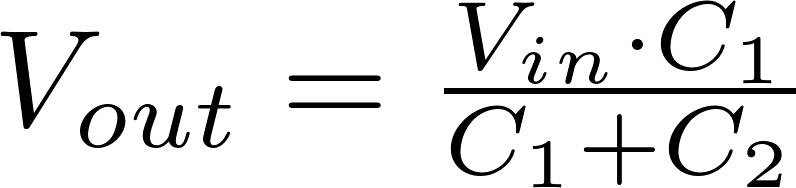The formulas for the other variables can be found using algebra: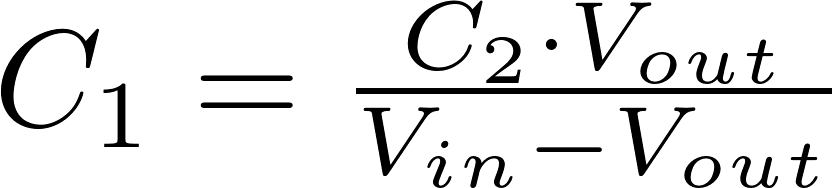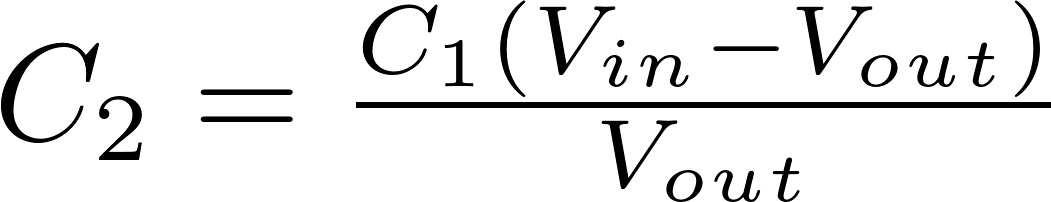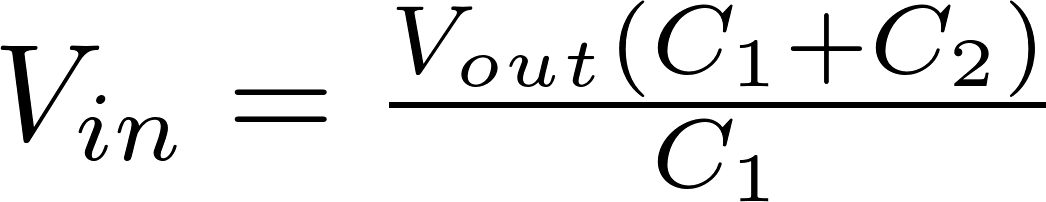V in is the input voltage and is measured in volt (V)
C is the symbol for capacitance and is measured in farad (F).
V out is the output voltage and is measured in volt (V)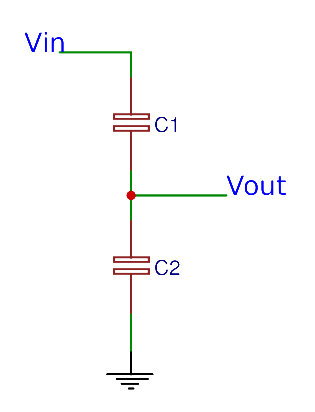### Calculator

Enter three values to calculate the remaining one.